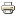# Roman GamesDate:
26-05-16
City:
Rome
Country:
Italy
Event format:
WorkshopThe Department of Computer, Control, and Management Engineering Antonio Ruberti (DIAG) hosts the fi rst of a series of one-day, informal meetings on Multiagent Optimization (game theory, optimization over networks, distributed computing, equilibrium problems and more).

The aim of these meetings is to bring together people in the roman region working in Multiagent Optimization but is open to contributions from anybody interested in this broad research area.
The meeting will take place on May the 26th, 2016 in the Aula Magna of DIAG, Via Ariosto 25, Roma. Participation is free but we kindly ask you to send us an mail at the address francisco.facchinei@uniroma1.it if you intend to come.

This first meeting has been organized by Francisco Facchinei (Universita di Roma La Sapienza) and Roberto Lucchetti (Politecnico di Milano).

10.00: Marco Scarsini (LUISS, Roma)
Hotelling Games on networks: Existence and eciency of equilibria

11.00: Stefano Leonardi (Universita di Roma La Sapienza, Roma)
Simple and ecient mechanisms for bayesian auctions

12.00: Roberto Lucchetti (Politecnico di Milano, Milano)
Strong Nash equilibria and mixed strategies

14.15: Marco Dall'Aglio (LUISS, Roma)
A geometrical framework for the fair allocation of divisible goods

15.15: Simone Sagratella (Universita di Roma La Sapienza, Roma)
Computing all solutions of Nash equilibrium problems with discrete strategy sets

Hotelling Games on networks: existence and eciency of equilibria
Gaetan Fournier, Tel Aviv University - School of Mathematical Sciences
Marco Scarsini, LUISS, Dipartimento di Economia e Finanza

We consider a Hotelling game where a nite number of retailers choose a location, given that their potential customers are distributed on a network. Retailers do not compete on price but only on location, therefore each consumer shops at the closest store. We show that when the number of retailers is large enough, the game admits a pure Nash equilibrium and we construct it. We then compare the equilibrium cost bore by the consumers with the cost that could be achieved if the retailers followed the dictate of a benevolent planner.
We perform this comparison in term of the induced price of anarchy, i.e., the ratio of the worst equilibrium cost and the optimal cost, and the induced price of stability, i.e., the ratio of the best equilibrium cost and the optimal cost. We show that, asymptotically in the number of retailers, these ratios are two and one, respectively.

Simple and ecient mechanisms for bayesian auctions
Stefano Leonardi, DIAG, Universita di Roma La Sapienza
We discuss the use of methods from the theory of algorithmic mechanism design and approximation algorithms to relax some of the assumptions and impossibility results in bayesian auction design. Problems that will be considered include prior-free mechanism
design, sequential posted-price mechanisms, double auctions and correlated valuations.

Strong Nash equilibria and mixed strategies
Eleonora Braggion, Roberto Lucchetti,
Dipartimento di Matematica, Politecnico di Milano

Nicola Gatti,
Dipartimento di Elettronica, Informazione e Bioningegneria, Politecnico di Milano,
Tuomas Saldhom,
Computer Science Department, Carnegie Mellon University, USA.

We consider strong Nash equilibria, in mixed strategies, for nite games. First, we analyze the twoplayer setting. Our main result, in its simplest form, states that if a game has a strong Nash equilibrium with full support, then the game is strictly competitive. Our
characterization enables us to design a strong-Nash-equilibrium- nding algorithm with complexity in Smoothed-P. So, this problem,that in general is computationally hard in the worst case, is generically easy. Hence, although the worst case complexity of finding
a strong Nash equilibrium is harder than that of nding a Nash equilibrium, once small perturbations are applied, nding a strong Nash is easier than nding a Nash equilibrium.
Next we switch to the setting with more than two players. We demonstrate that a strong Nash equilibrium can exist in which an outcome that is strictly Pareto dominated by a Nash equilibrium occurs with positive probability. Finally, we prove that the set of games
having a strong Nash equilibrium where at least one player puts positive probability on at least two pure strategies is extremely small in any respect (in Baire sense, of null measure, -porous, sparse).

A geometrical framework for the fair allocation of divisible goods
Marco Dall'Aglio, Camilla Di Luca and Lucia Milone,
LUISS, Dipartimento di Economia e Finanza

We characterize the maxmin partition of one or more in nitely divisble goods disputed among a nite number of agents with idiosyncratic preferences. In particular:
1. We provide a two-sided inequality for the optimal partition value The result improves on existing results by Elton et al. (1986) and Legut (1988).
2. We describe an algorithm, based on the projected subgradient method (Boyd and Vandenberghe, Convex Optimization, 2003), that computes approximations, up to any threshold, of the optimal value and the optimal partition.
3. We consider, as a special case, the division of a nite number of homogeneous divisible items among three agents We characterize the optimal allocations and we develop an exact algorithm for its search.

Computing all solutions of Nash equilibrium problems with discrete strategy sets
Simone Sagratella, DIAG, Universita di Roma La Sapienza, Roma
The Nash equilibrium problem is a widely used tool to model non-cooperative games. Many solution methods have been proposed in the literature to compute solutions of Nash equilibrium problems with continuous strategy sets, but, besides some speci c methods for
some particular applications, there are no general algorithms to compute solutions of Nash equilibrium problems in which the strategy set of each player is assumed to be discrete.  We de ne a branching method to compute the whole solution set of Nash equilibrium
problems with discrete strategy sets. This method is equipped with a procedure that, by fixing variables, e effectively prunes the branches of the search tree. Furthermore, we propose a preliminary procedure that by shrinking the feasible set improves the performances of the branching method when tackling a particular class of problems. Moreover, we prove existence of equilibria and we propose an extremely fast Jacobi-type method which leads to one equilibrium for a new class of Nash equilibrium problems with discrete strategy sets. Our numerical results show that all the proposed algorithms work very well in practice.

Developed by Paolo Gittoi